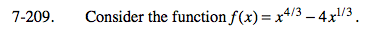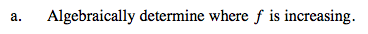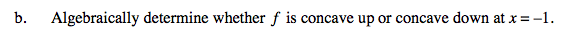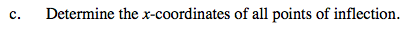### Home > CALC > Chapter 7 > Lesson 7.4.4 > Problem7-209

7-209.Where is f '(x) positive?Solve f ''(x) > 0 and f ''(x) < 0.An inflection point occurs where f ''(x) undergoes a sign change.
Sometimes that happens where f ''(x) = 0 and sometimes where f ''(x) = DNE.